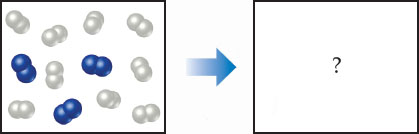# Problem: In the following diagram, the white spheres represent hydrogen atoms and the blue spheres represent nitrogen atoms.The two reactants combine to form a single product, ammonia, NH3, which is not shown. Write a balanced chemical equation for the reaction. Based on the equation and the contents of the left (reactants) box how many molecules should be shown in the right (products) box?

###### FREE Expert Solution

When balancing chemical equations the number of atoms of each element must be the same for both sides (reactants and products) of the chemical equation.

Complete reaction: H2 + N2 → NH3

79% (110 ratings)###### Problem Details

In the following diagram, the white spheres represent hydrogen atoms and the blue spheres represent nitrogen atoms.The two reactants combine to form a single product, ammonia, NH3, which is not shown. Write a balanced chemical equation for the reaction. Based on the equation and the contents of the left (reactants) box how many molecules should be shown in the right (products) box?